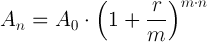# Compound Interest Formula

Compound interest calculation formula with examples.

## Compound interest calculation formula

### Future value calculation

The future amount after n years An is equal to the initial amount A0 times one plus the annual interest rate r divided by the number of compounding periods in a year m raised to the power of m times n:An is the amount after n years (future value).

A0 is the initial amount (present value).

r is the nominal annual interest rate.

m is the number of compounding periods in one year.

n is the number of years.

#### Example #1:

Calculate the future value after 10 years present value of \$3,000 with annual interest of 4%.

Solution:

A0 = \$3,000

r = 4% = 4/100 = 0.04

m = 1

n = 10

A10 = \$3,000·(1+0.04/1)(1·10) = \$4,440.73

#### Example #2:

Calculate the future value after 8 years present value of \$40,000 with annual interest of 3% compounded monthly.

Solution:

A0 = \$40,000

r = 3% = 3/100 = 0.03

m = 12

n = 8

A8 = \$40,000·(1+0.03/12)(12·8) = \$50,834.74

#### Example #3:

Calculate the future value after 8 years present value of \$50,000 with annual interest of 4% compounded monthly.

Solution:

A0 = \$50,000

r = 4% = 4/100 = 0.04

m = 12

n = 8

A8 = \$50,000·(1+0.04/12)(12·8) = \$68,819.76

#### Example #4:

Calculate the future value after 8 years present value of \$70,000 with annual interest of 5% compounded monthly.

Solution:

A0 = \$70,000

r = 5% = 5/100 = 0.05

m = 12

n = 8

A8 = \$70,000·(1+0.05/12)(12·8) = \$104,340.98

Compound interest calculator ►